﻿ Java 缺失的特性：操作符重載_ZenDei技術網路在線

# Java 缺失的特性：操作符重載``本文介紹了什麼是操作符重載、為什麼需要操作符重載、如何在Java中實現操作符重載以及一些建議。``

``private static final BigInteger BI_2 = BigInteger.valueOf(2);``

``BigInteger res = a.multiply(a).subtract(BI_2.multiply(a).multiply(b)).add(b.multiply(b));``

``BigInteger res = a * a - BI_2 * a * b + b * b;``

1. 代碼寫起來更簡單，不容易出錯
2. 代碼更容易閱讀，不會一堆括弧嵌套

Manifold 是將每個算數操作符的重載，映射到特定名稱的函數。例如你在某個類 A 中定義了 plus(B) 的方法，那麼這個類就可以使用 a + b 代替 a.plus(b) 進行調用。具體的映射關係為：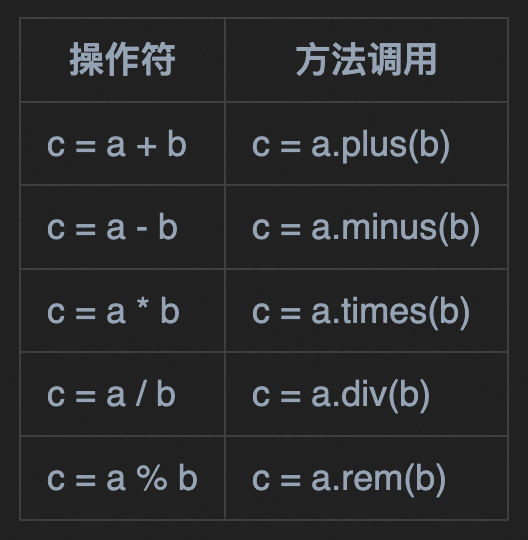—— 用過 Kotlin 的同學應該會會心一笑，這就是模仿的 Kotlin 的操作符重載。

``````
public class Num {

private final int v;

public Num(int v) {
this.v = v;
}

public Num plus(Num that) {
return new Num(this.v + that.v);
}

public Num minus(Num that) {
return new Num(this.v - that.v);
}

public Num times(Num that) {
return new Num(this.v * that.v);
}
}``````

``````
Num a = new Num(1);
Num b = new Num(2);

Num c = a + b - a;``````

Manifold 在編譯期處理之後，會變成：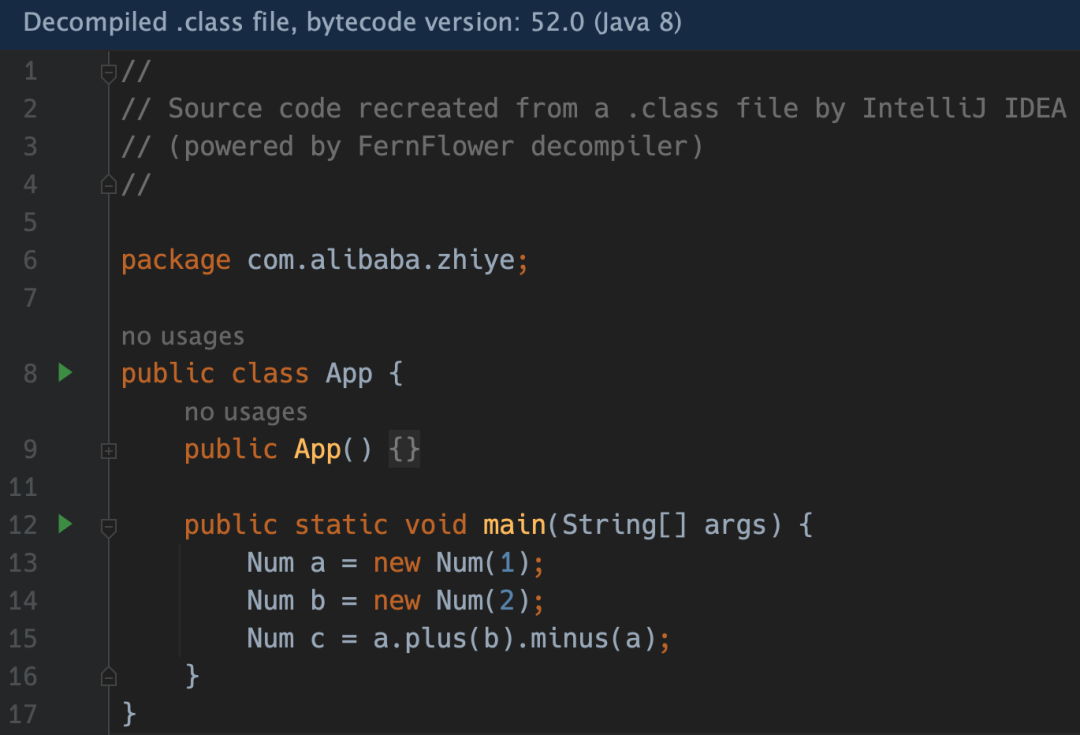``Num c = a + a * b - b;``
Manifold 處理之後，則是：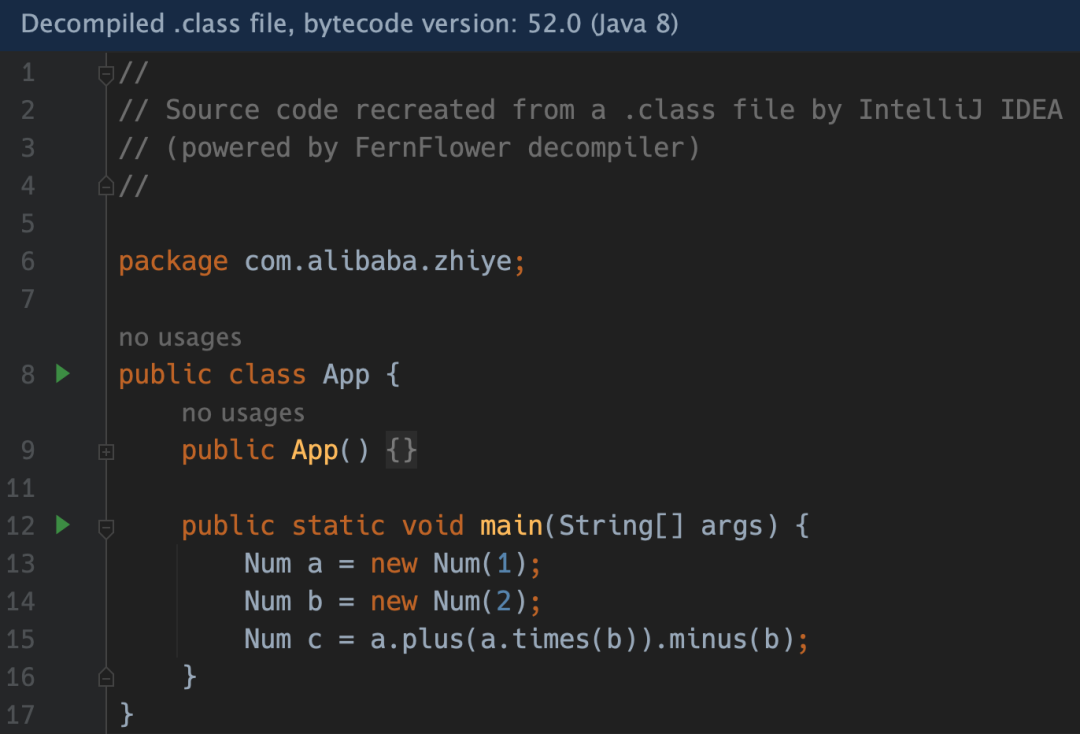``````public class Num {

...

public Num plus(Num that) {
return new Num(this.v + that.v);
}

public Num plus(int i) {
return new Num(v + i);
}
}``````

``Num c = a + 1 + b;``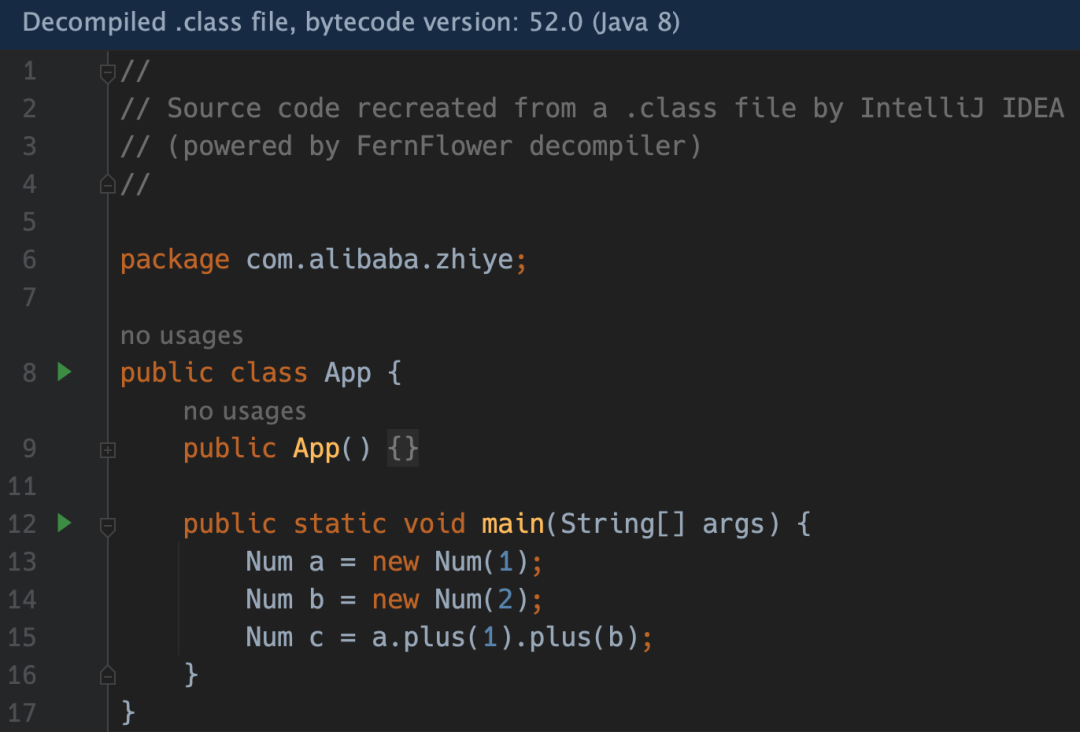Java 對原始類型中的數值支持複合賦值，即 +=、-= 這些，Manifold 也支持：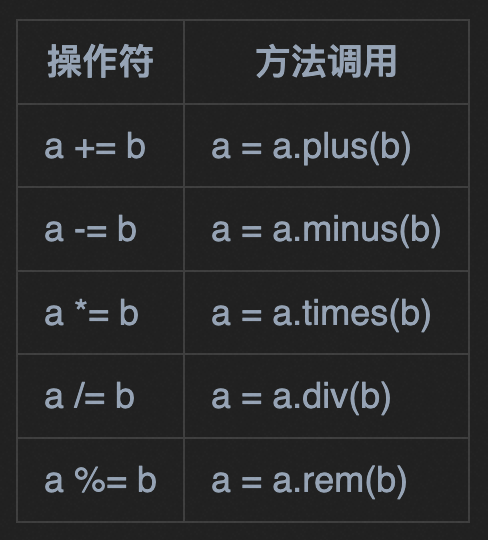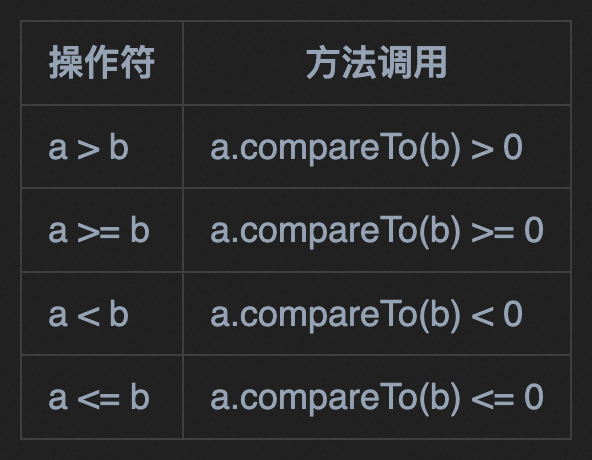``````
public class Num implements Comparable<Num> {

...

@Override
public int compareTo(Num that) {
return this.v - that.v;
}
}``````

``````
Num a = new Num(1);
Num b = new Num(2);

if (a > b) {
System.out.println("a > b");
}

if (a < b) {
System.out.println("a < b");
}``````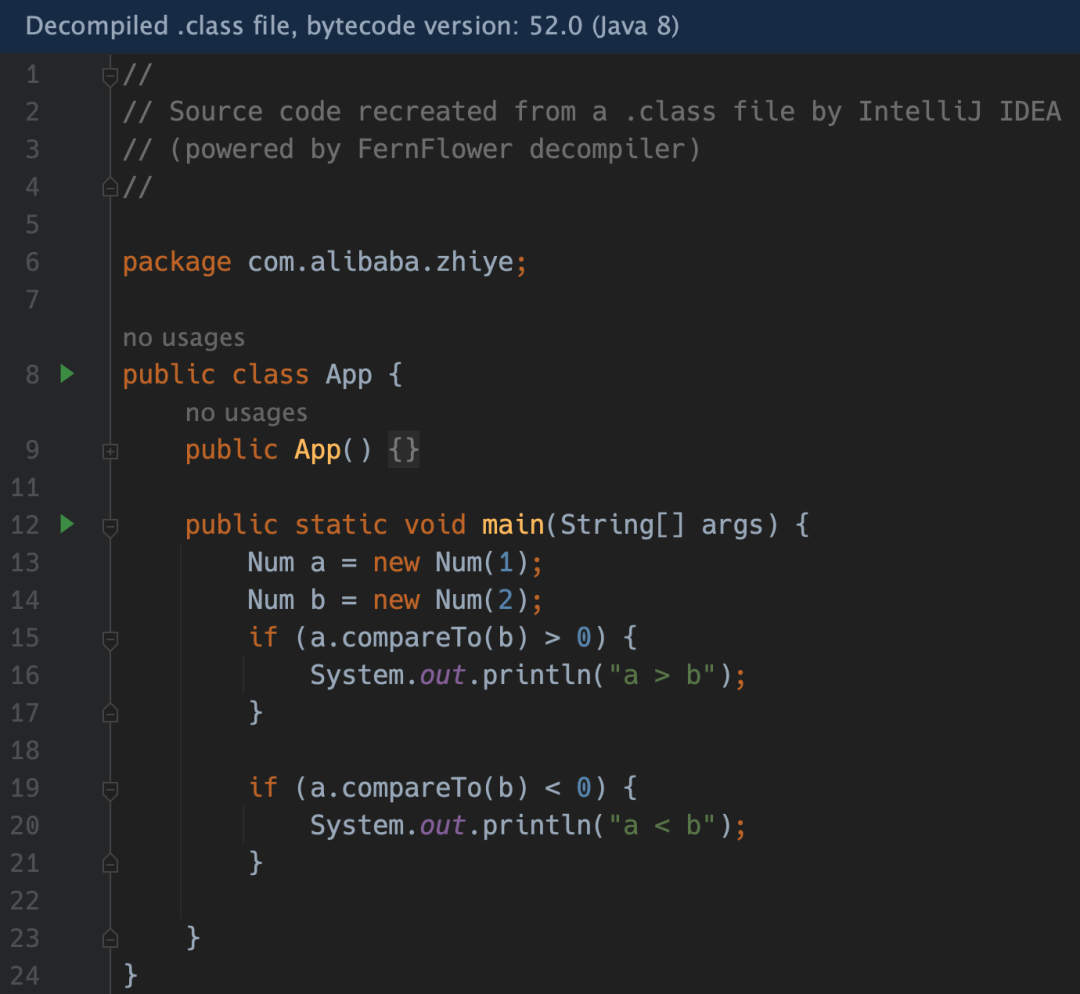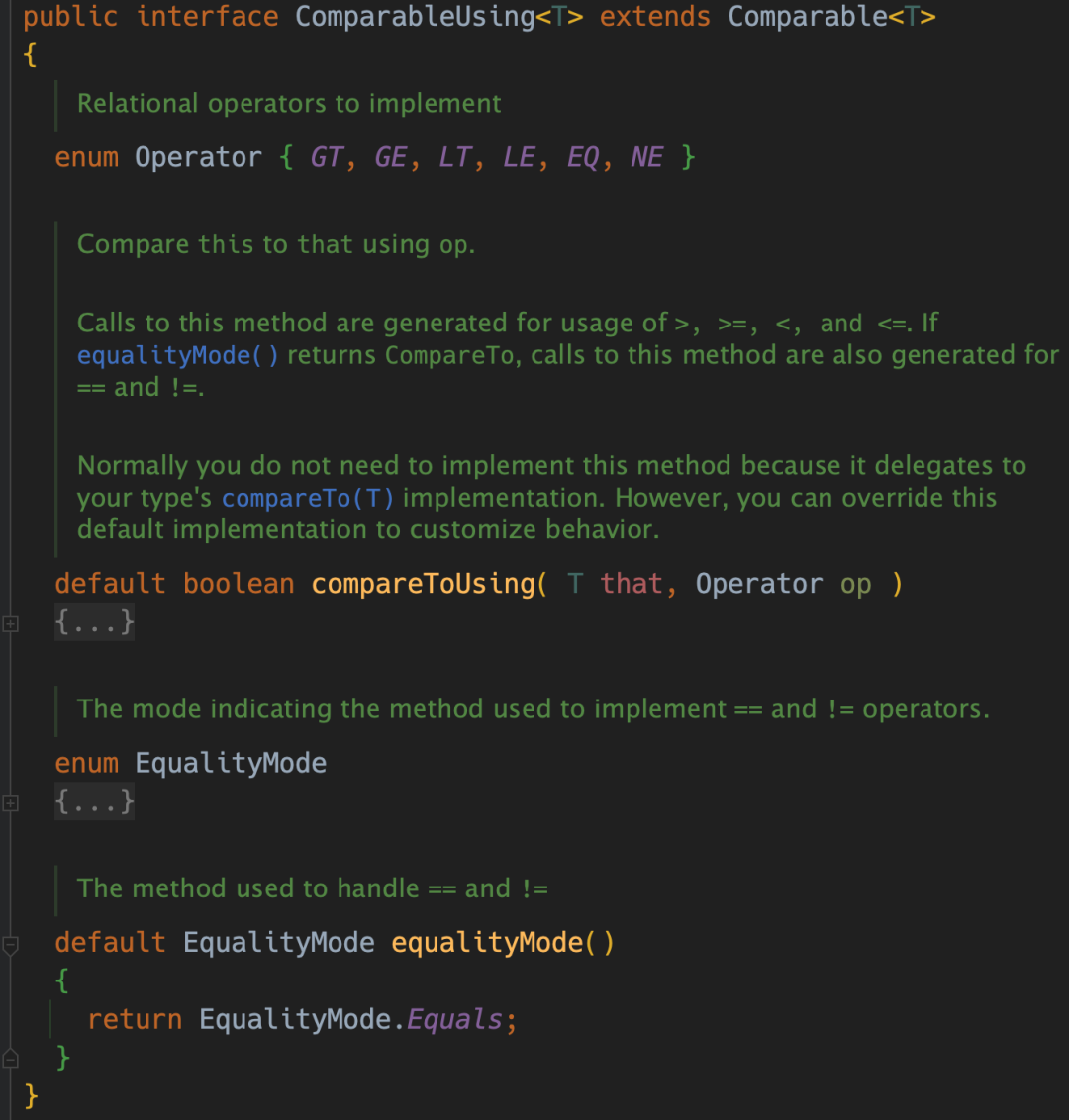ComparableUsing<T> 繼承了 Comparable<T> 介面，並且添加了兩個方法，compareToUsing 和 equalityMode。查看 comparableUsing 的預設實現：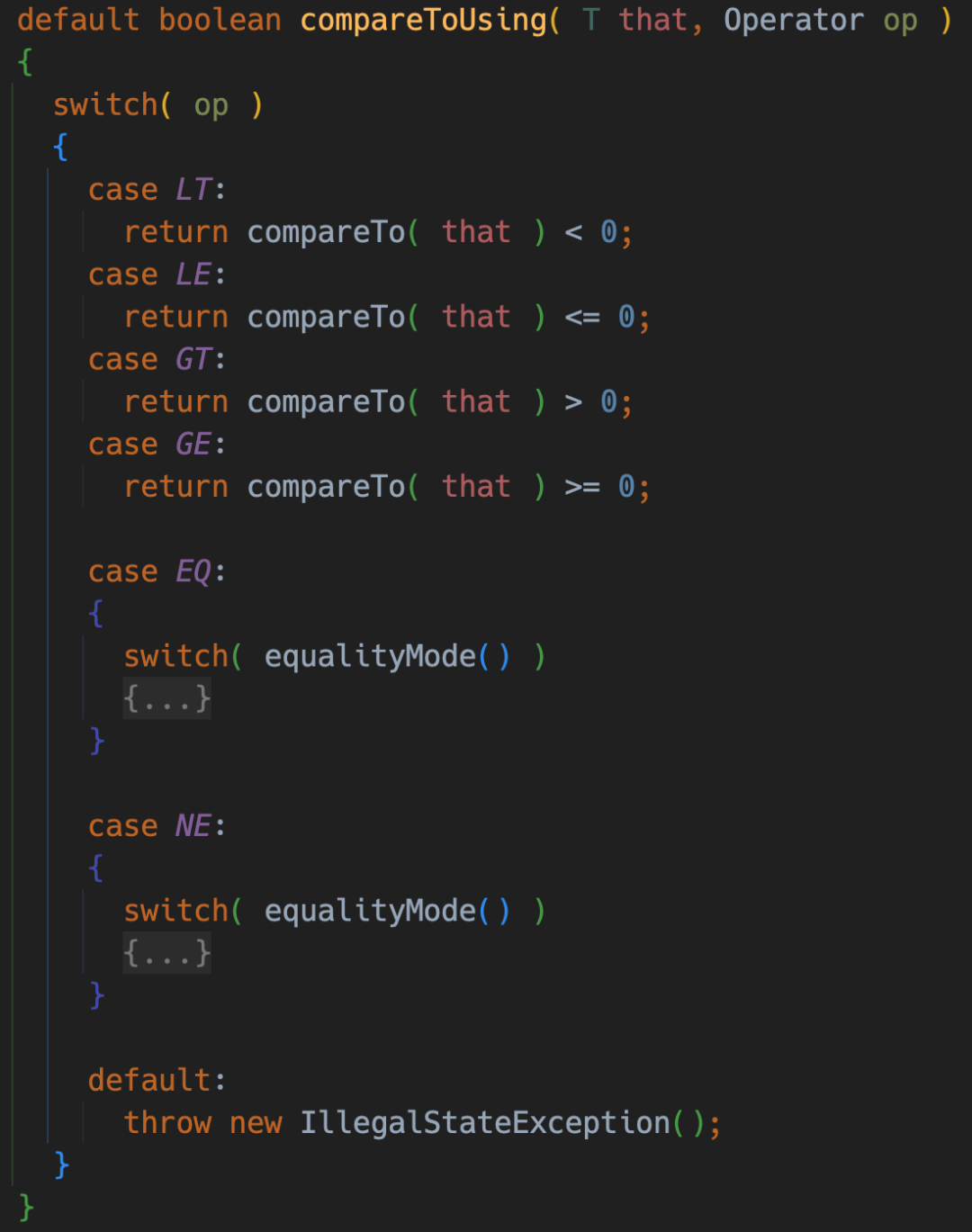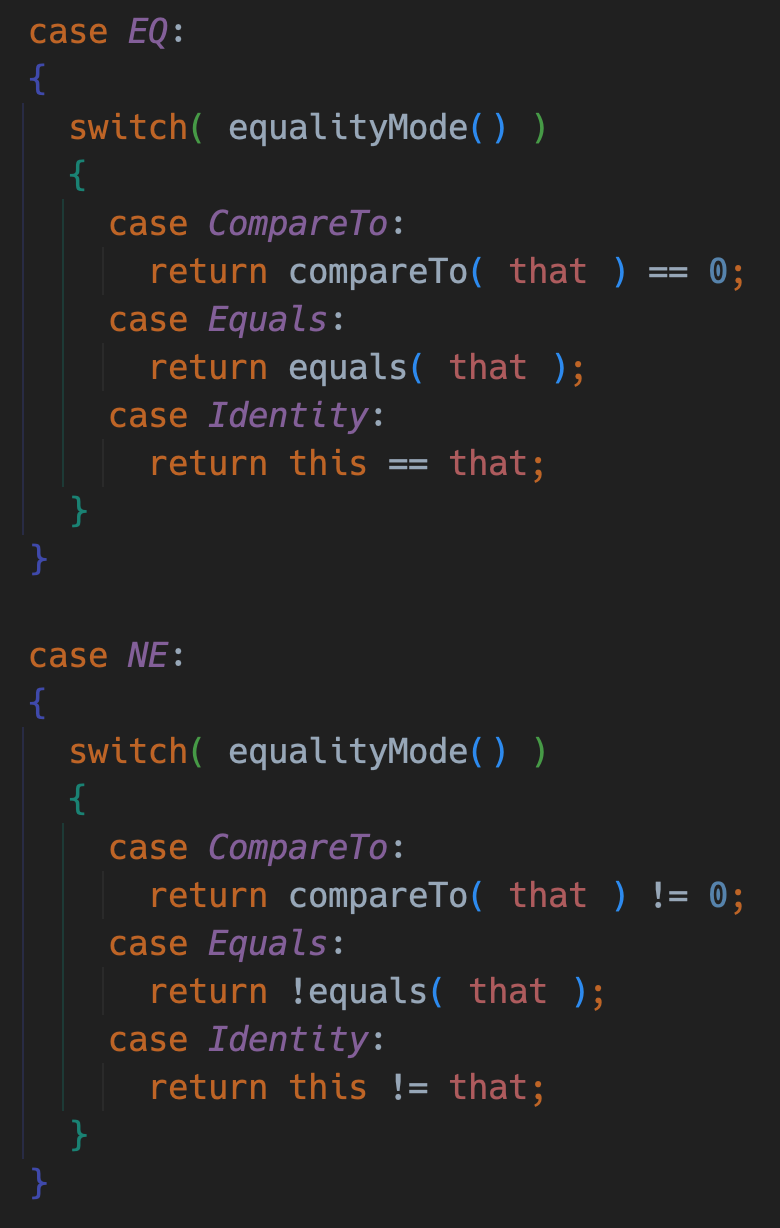• 如果是
EqualityMode.CompareTo
，則
==
和
!=
的重載分別對應的是
compareTo
方法返回值為 0 和 非0 的情況。
• 如果是
EqualityMode.Equals
，則
==
和
!=
的重載分別對應的是
equals
方法返回值為
true
和
false
的情況。
• 如果是
EqualityMode.Identity
，那使用的是 Java 的預設實現，即比較對象的引用地址是否相同。

``````
public class Num implements ComparableUsing<Num> {

...

@Override
public int compareTo(Num that) {
return this.v - that.v;
}

@Override
public boolean equals(Object obj) {
if (this == obj) { return true; }

if (obj instanceof Num) {
Num that = (Num) obj;
return this.v == that.v;
}

return false;
}

@Override
public int hashCode() {
return Objects.hash(v);
}
}``````

``````
Num a = new Num(1);
Num b = new Num(1);

if (a == b) {
System.out.println("a == b");
}

if (a != b) {
System.out.println("a != b");
}``````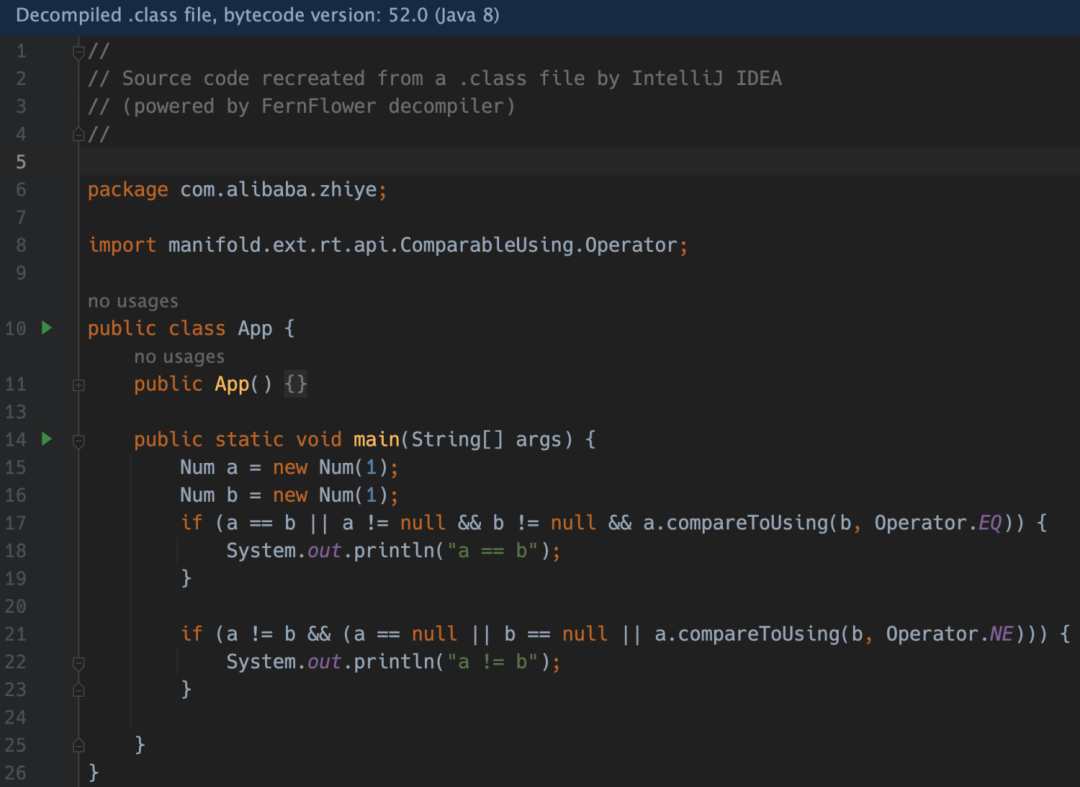Amazing！我們終於實現了 N 年前的夢想，讓 == 和 != 是使用 equals 方法的邏輯進行比較，而不是比較引用地址。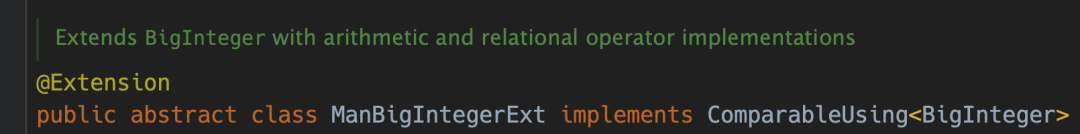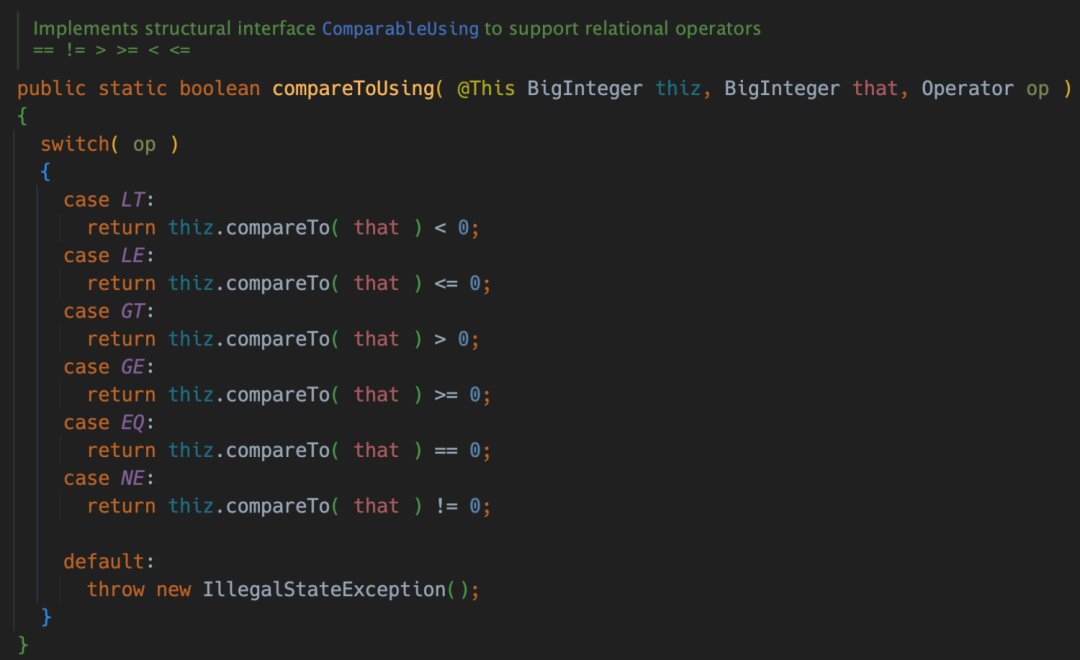Java 對數組是支持索引操作符的，比如 nums[i] 是訪問數組索引為 i 的元素，nums[i] = n 是對數組索引為 i 的位置進行賦值。但對 List 和 Map，Java 說 “不好意思，因為我是 Java，這個支持不了”。所以 Manifold 又出手了，讓你不再只能羡慕其他語言。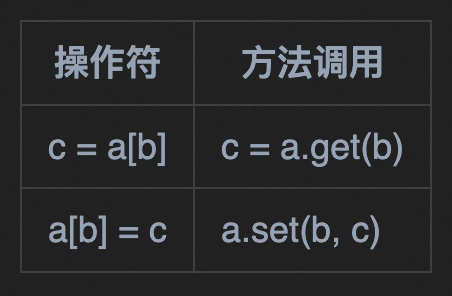``````
@Extension
public class MapExt {

public static <K, V> V set(@This Map<K, V> map, K key, V value) {
return map.put(key, value);
}
}``````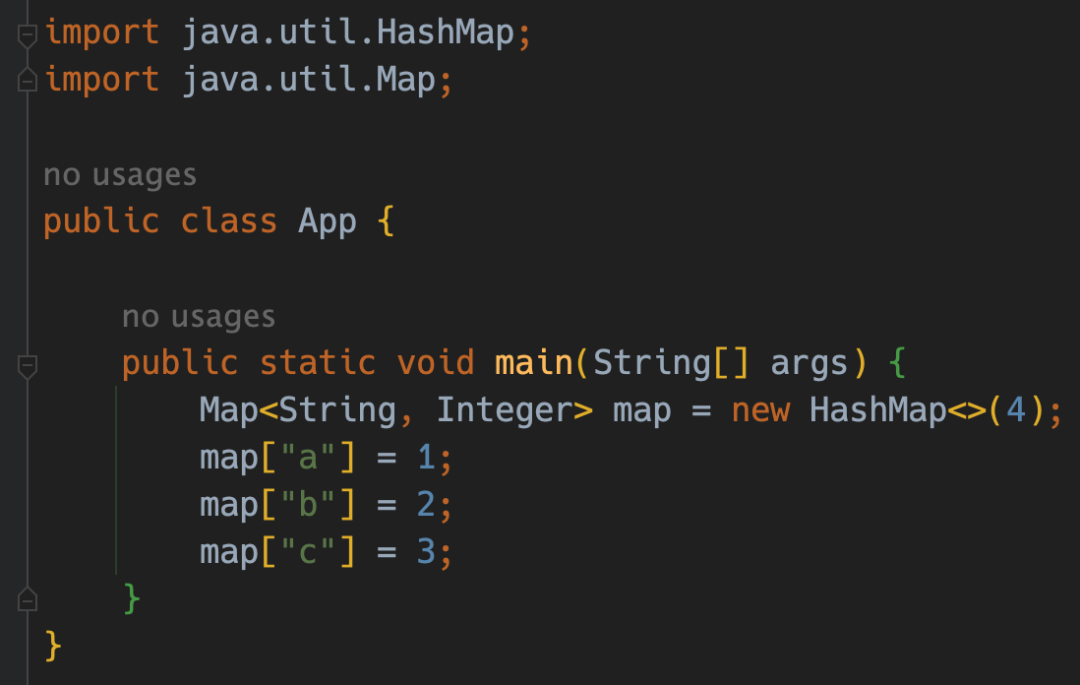``````
int[] nums = {1, 2, 3};
int value = nums = 10;``````

``````
List<String> list = Arrays.asList("a", "b", "c");
String value = list = "A";``````

Manifold 處理之後，代碼會變成：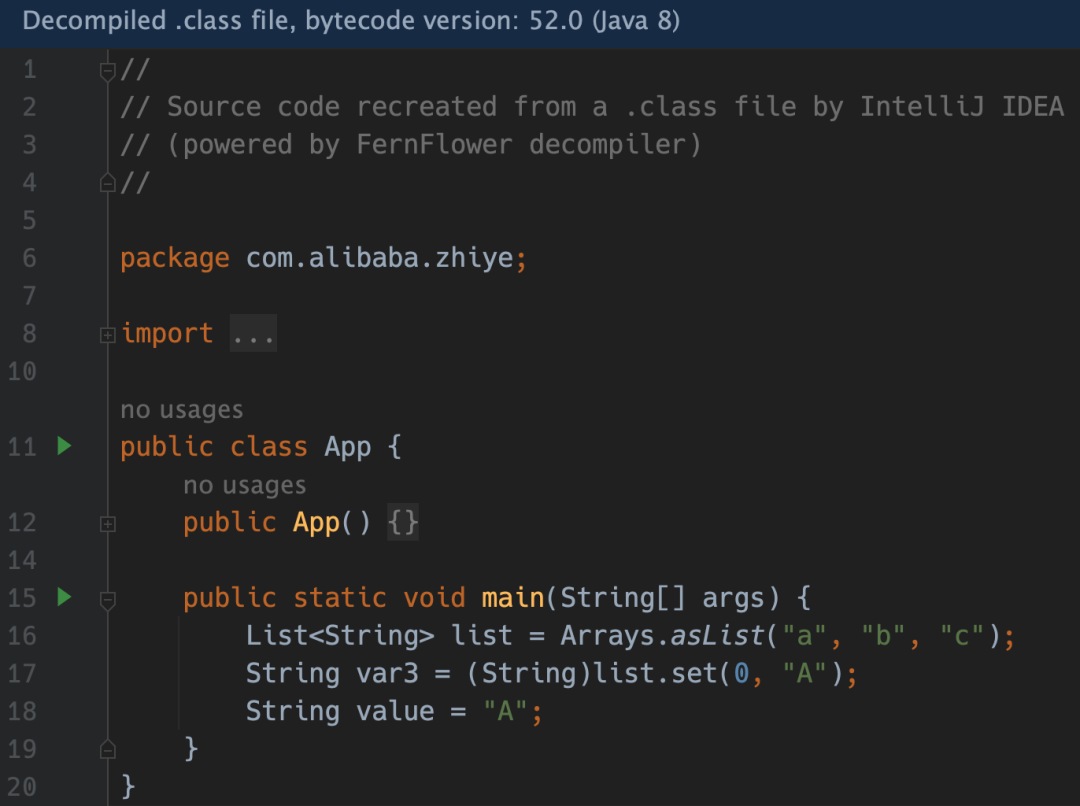Manifold 還提供一種非常有意思的功能：單位操作符。顧名思義，就是我們可以在代碼中提供“單位”功能。比如下麵這種代碼：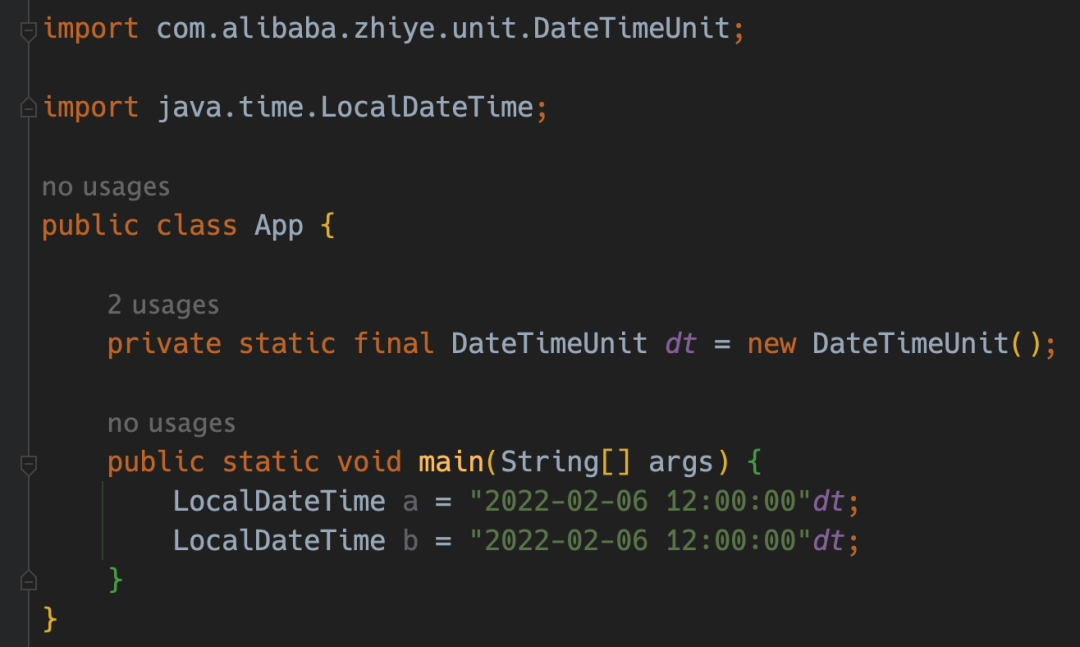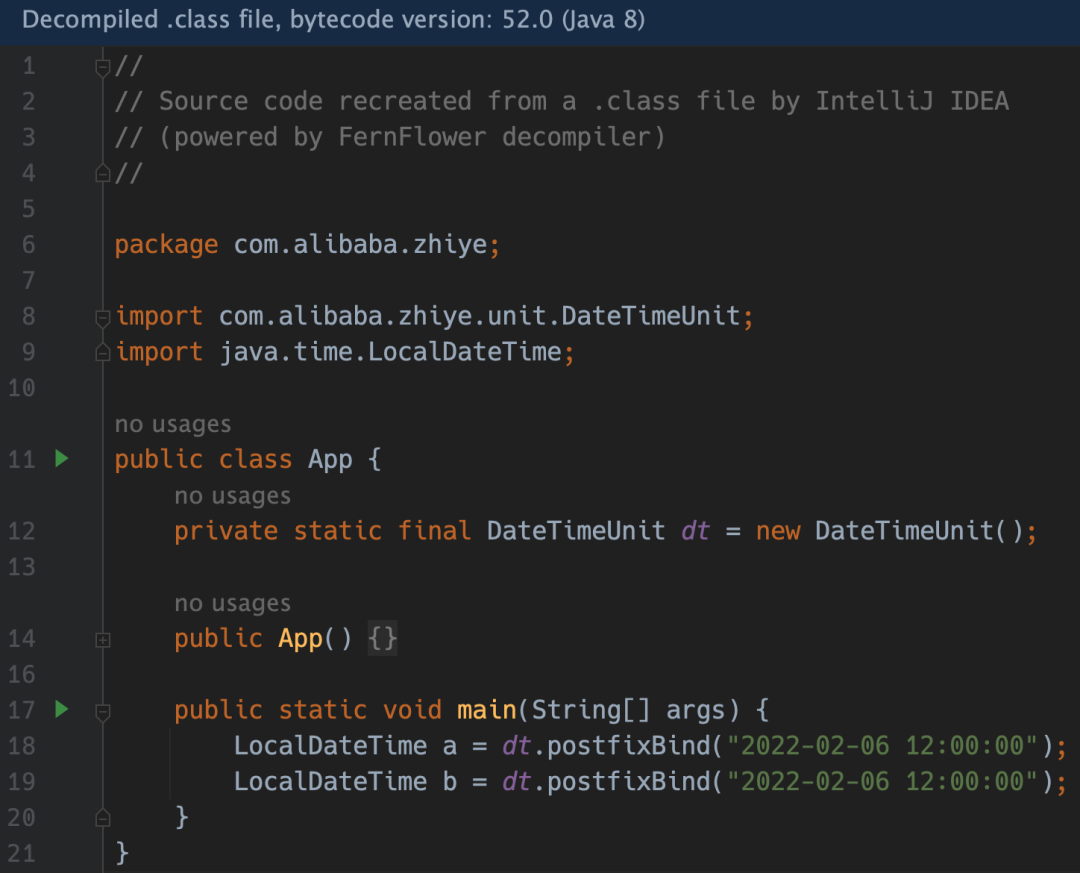``````
public class DateTimeUnit {

private static final
DateTimeFormatter FORMATTER = DateTimeFormatter.ofPattern("yyyy-MM-dd HH:mm:ss");

public LocalDateTime postfixBind(String value) {
return LocalDateTime.parse(value, FORMATTER);
}
}``````

postfixBind 表示這個單位是“尾碼單位”，就是你看到的 "xxx"dt，dt 在 "xxx" 的後面。Manifold 同時也支持“首碼單位”，對應的方法是 prefixBind，比如：

``````
public class DateTimeUnit {

...

public LocalDateTime prefixBind(String value) {
return LocalDateTime.parse(value, FORMATTER);
}
}``````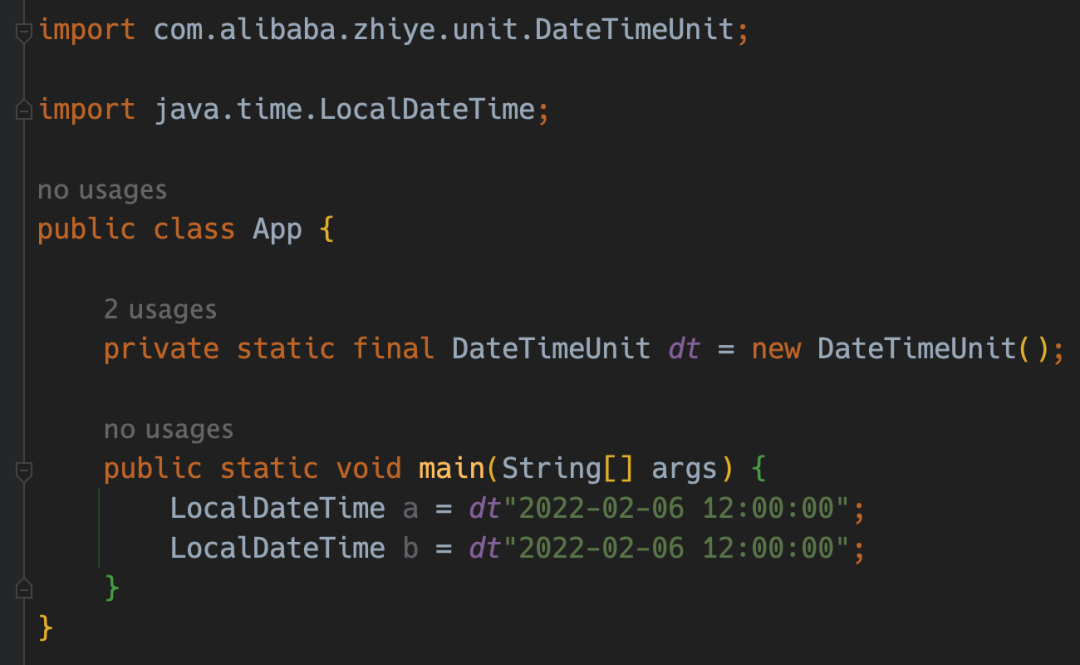Amazing！有了“單位”功能，我們就可以做出很多實用的“字面量”功能。比如定義 BigInteger 的“單位”：
``````
public class BigIntegerUnit {

public BigInteger postfixBind(Integer value) {
return BigInteger.valueOf(value);
}

public BigInteger postfixBind(String value) {
return new BigInteger(value);
}
}``````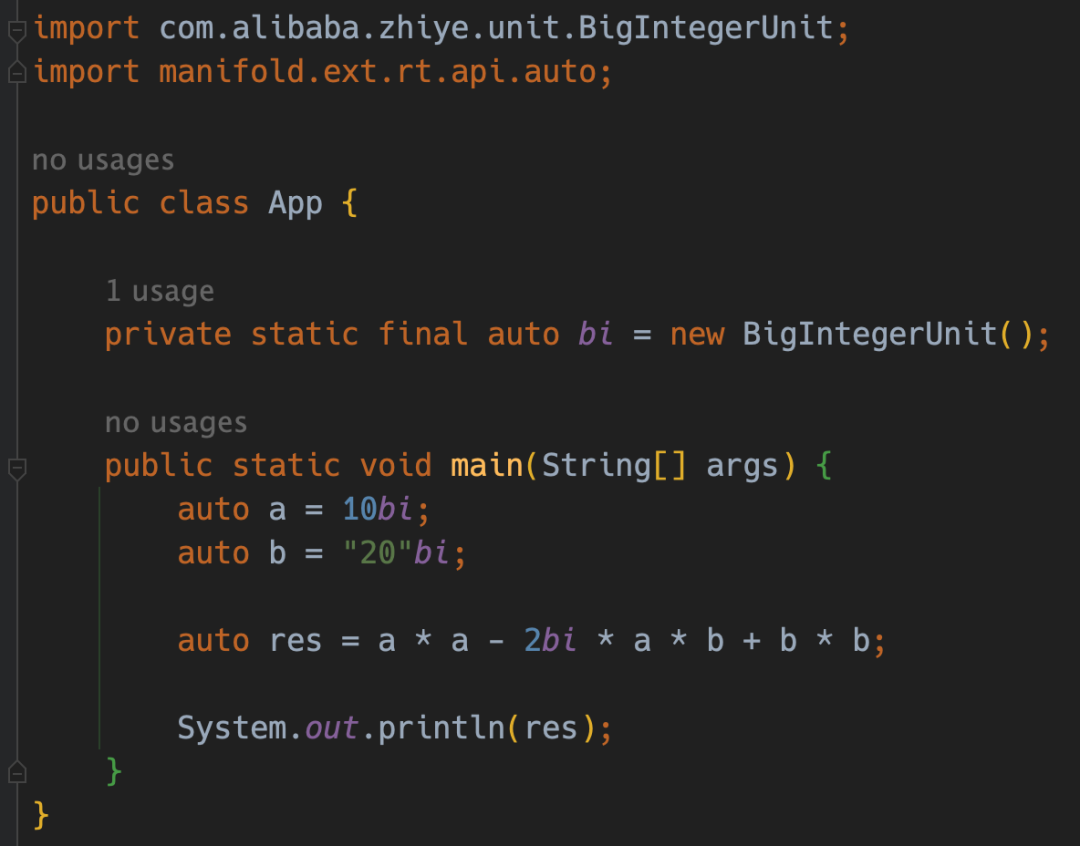``````public class MapEntryBuilder {

public <K> EntryKey<K> postfixBind(K key) {
return new EntryKey<>(key);
}

public static class EntryKey<K> {

private final K key;

public EntryKey(K key) {
this.key = key;
}

public <V> Map.Entry<K, V> prefixBind(V value) {
return new AbstractMap.SimpleImmutableEntry<>(key, value);
}
}
}``````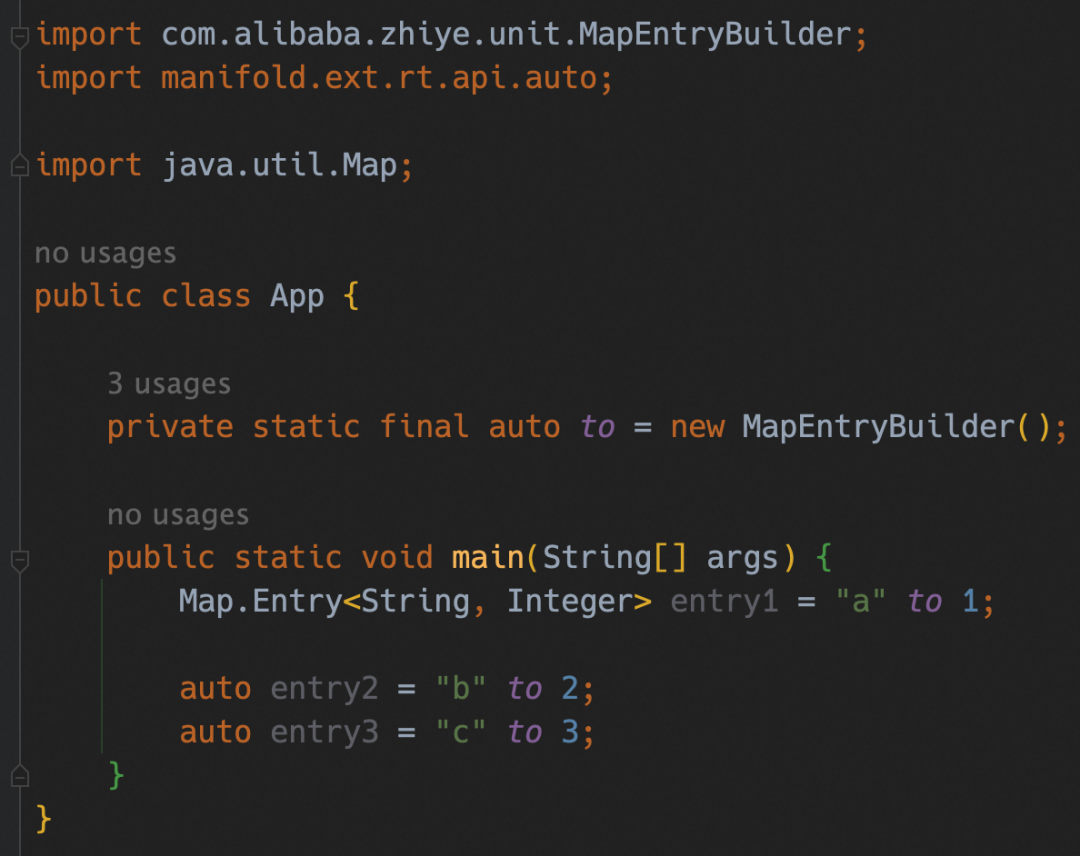``````
@Extension
public class MapExt {

@Extension
@SafeVarargs
public static <K, V> Map<K, V> of(Map.Entry<K, V>... entries) {
Map<K, V> map = new LinkedHashMap<>(entries.length);

for (Map.Entry<K, V> entry : entries) {
map.put(entry.getKey(), entry.getValue());
}

return Collections.unmodifiableMap(map);
}
}``````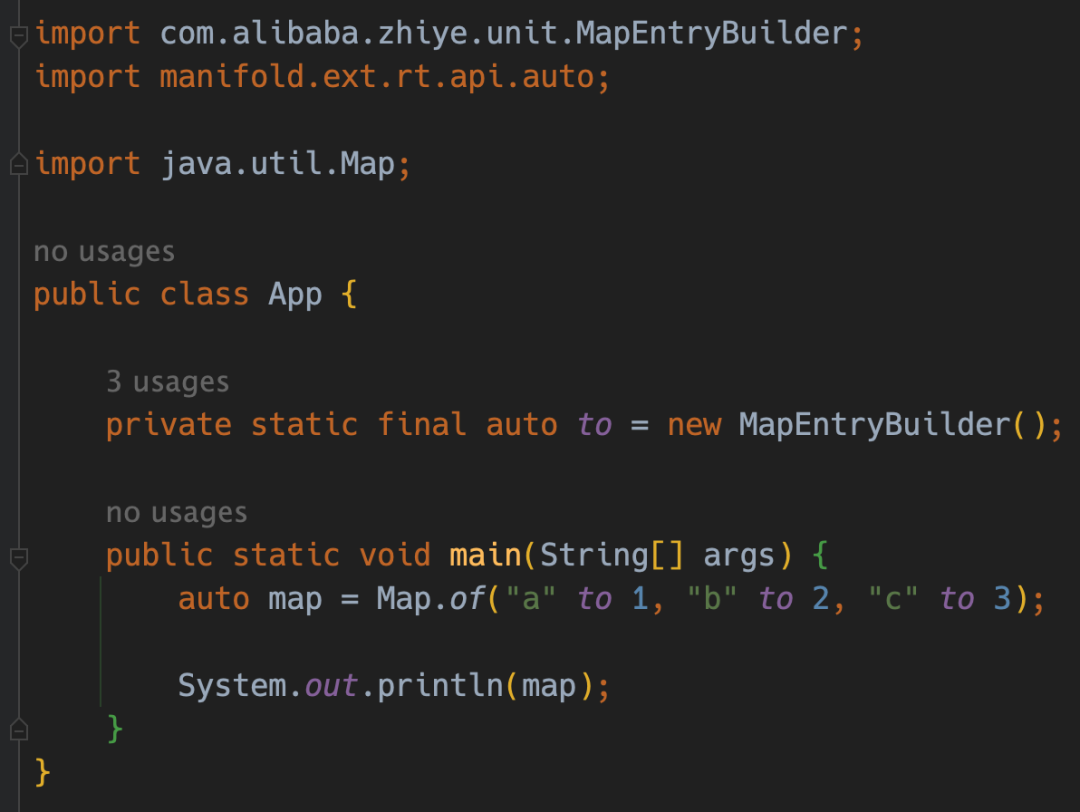Java 一直以來都不支持操作符重載，肯定是有其原因的。作為一門之前主打企業應用開發的語言，確實操作符重載不是必要的。但隨著硬體的發展，我們也看到 Java 越來越多的出現在數據科學/高性能計算的領域，同時 Java 也開始嘗試提供值類型：Project Valhalla。所以，也許在不久後的將來，隨著值類型在計算方面的廣泛應用，在 Java 中提供操作符重載的呼聲會越來越高，進而被 JCP 採納。而 Manifold 作為先驅者，提前讓我們可以體驗未來的 Java，幸甚至哉！

https://github.com/manifold-systems/manifold

https://openjdk.org/jeps/8277163• 本文介紹在Anaconda環境下，安裝Python中的一個高級地理空間數據分析庫whitebox的方法。 首先，我們打開“Anaconda Prompt (Anaconda)”軟體。 隨後，將彈出如下所示的命令輸入視窗。 在上述彈出的命令輸入視窗中，輸入以下代碼： conda install -c ...
• tar 備忘清單 IT寶庫網整理的關於 tar 常用命令的快速參考備忘單。入門，為開發人員分享快速參考備忘單。 開發速查表大綱 入門 介紹 選項 創建一個 tar 格式的壓縮文件 創建壓縮後的 tar.gz 存檔文件 生成壓縮率更高的 tar.bz2 文件 解壓縮 tar 文件 解壓縮 tar.gz ...
• 前言 互動直播是實現很多熱門場景的基礎，例如直播帶貨、秀場直播，還有類似抖音的直播 PK等。本文是由聲網社區的開發者“小猿”撰寫的Flutter基礎教程系列中的第二篇，他將帶著大家用一個小時，利用聲網 Flutter SDK 實現視頻直播、發評論、送禮物等基礎功能。 開發一個跨平臺的的直播的功能需要 ...
• 前後端分離下EasyExcel的使用 項目環境:SpringBoot+Vue 依賴導入 <!--easyexcel--> <dependency> <groupId>com.alibaba</groupId> <artifactId>easyexcel</artifactId> <version>3 ...
• 小梅哥的這個ZYNQ開發板上的DDR3位於PS側，PL側想要使用DDR3作為緩存的話，得通過HP介面來與PS側的DDR3控制進行通信。 本次實驗在小梅哥OV5640工程的基礎上，通過修改VDMA的S2MM端的模塊而來的。 將VMDA的幀緩存區設為1，關閉幀同步的功能後，其實和DMA差不多。 一、需要 ...
• 🎈 開啟多個 ws 服務失敗 正常情況下，如果你想開啟多個 websocket 服務的話 只要在一個文件中，輸入 new Worker 兩次，監聽不同埠，使用 Worker::runAll() 命令即可 但是你會發現在在 windows 中無法在一個文件中同時監聽兩個 websocket 服務， ...
• 來源：https://liuchenyang0515.blog.csdn.net/article/details/121049426 1. 雙重校驗鎖單例（DCL） public class Singleton { private static volatile Singleton singleto ...
• 訂單業務一直都是系統研發中的核心模塊，訂單的產生過程，與系統中的很多模塊都會高度關聯，比如賬戶體系、支付中心、運營管理等，即便單看訂單本身，也足夠的複雜； ...